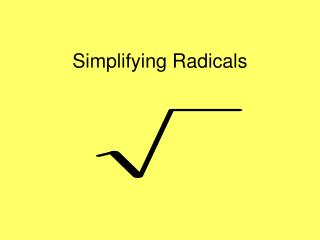DownloadDownload PresentationDownload Presentation- - - - - - - - - - - - - - - - - - - - - - - - - - - E N D - - - - - - - - - - - - - - - - - - - - - - - - - - -
##### Presentation Transcript

2. Perfect Squares 64 225 1 81 256 4 100 289 9 121 16 324 144 25 400 169 36 196 49 625

3. Simplify = 2 = 4 = 5 This is a piece of cake! = 10 = 12

4. Perfect Square Factor * Other Factor Simplify = = = = LEAVE IN RADICAL FORM = = = = = =

5. Perfect Square Factor * Other Factor Simplify = = = = LEAVE IN RADICAL FORM = = = = = =

7. Simplify each expression

8. Perfect Square Factor * Other Factor Simplify = = = = LEAVE IN RADICAL FORM = = = = = =

9. Simplify each expression

10. Simplify each expression

12. Multiply and then simplify

13. Dividing Radicals To divide radicals: divide the coefficients, divide the radicands if possible, and rationalize the denominator so that no radical remains in the denominator

14. That was easy!

15. This cannot be divided which leaves the radical in the denominator. We do not leave radicals in the denominator. So we need to rationalize by multiplying the fraction by something so we can eliminate the radical in the denominator. 42 cannot be simplified, so we are finished.

16. This can be divided which leaves the radical in the denominator. We do not leave radicals in the denominator. So we need to rationalize by multiplying the fraction by something so we can eliminate the radical in the denominator.

17. This cannot be divided which leaves the radical in the denominator. We do not leave radicals in the denominator. So we need to rationalize by multiplying the fraction by something so we can eliminate the radical in the denominator. Reduce the fraction.

18. Simplify = X = Y3 = P2X3Y = 2X2Y = 5C4D10

19. Simplify = = = =

20. = = ? = =